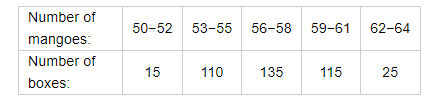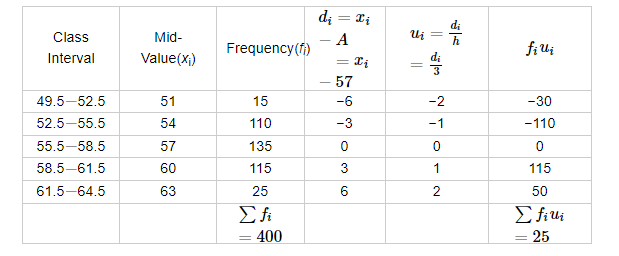Deepak Scored 45->99%ile with Bounce Back Crack Course. You can do it too!

# In a retail market, fruit vendors were selling mangoes kept in packing boxes.

Question:

In a retail market, fruit vendors were selling mangoes kept in packing boxes. These boxes contained varying number of mangoes. The following was the distribution of mangoes according to the number of boxes.Find the mean number of mangoes kept in a packing box. Which method of finding the mean did you choose?

Solution:

The given series is an inclusive series. Firstly, make it an exclusive series.Let the assumed mean be A = 57 and h = 3.

We know that mean, $\bar{X}=A+h\left(\frac{1}{N} \sum f_{i} u_{i}\right)$

Now, we have $N=\sum f_{i}=400, \sum f_{i} u_{i}=25, h=3$ and $A=57$.

Putting the values in the above formula, we have

$\bar{X}=A+h\left(\frac{1}{N} \sum f_{i} u_{i}\right)$

$=57+3\left(\frac{1}{400} \times(25)\right)$

$=57+\frac{75}{400}$

$=57+0.187$

$=57.187$

Hence, the mean is approximately $57.19$.